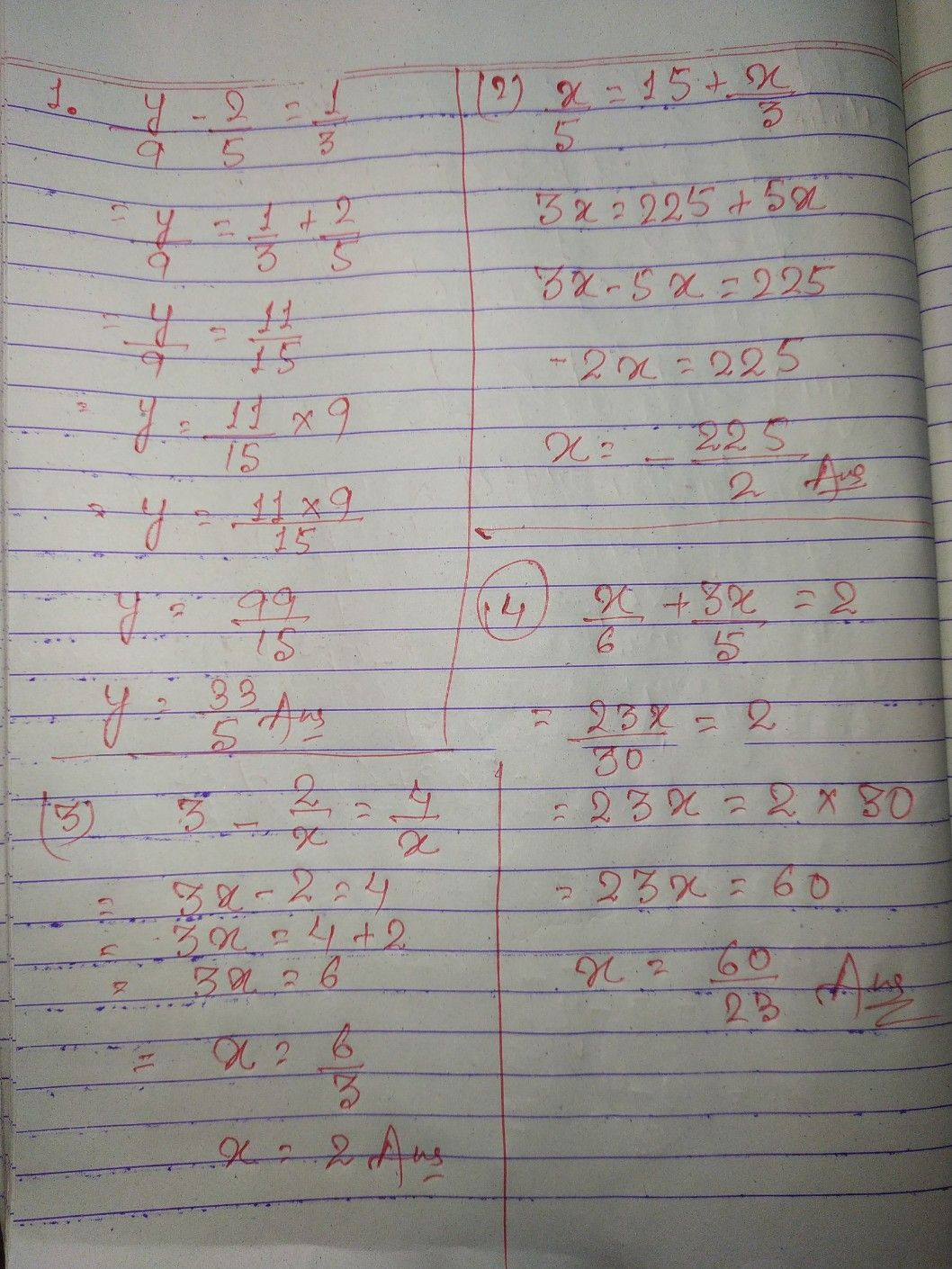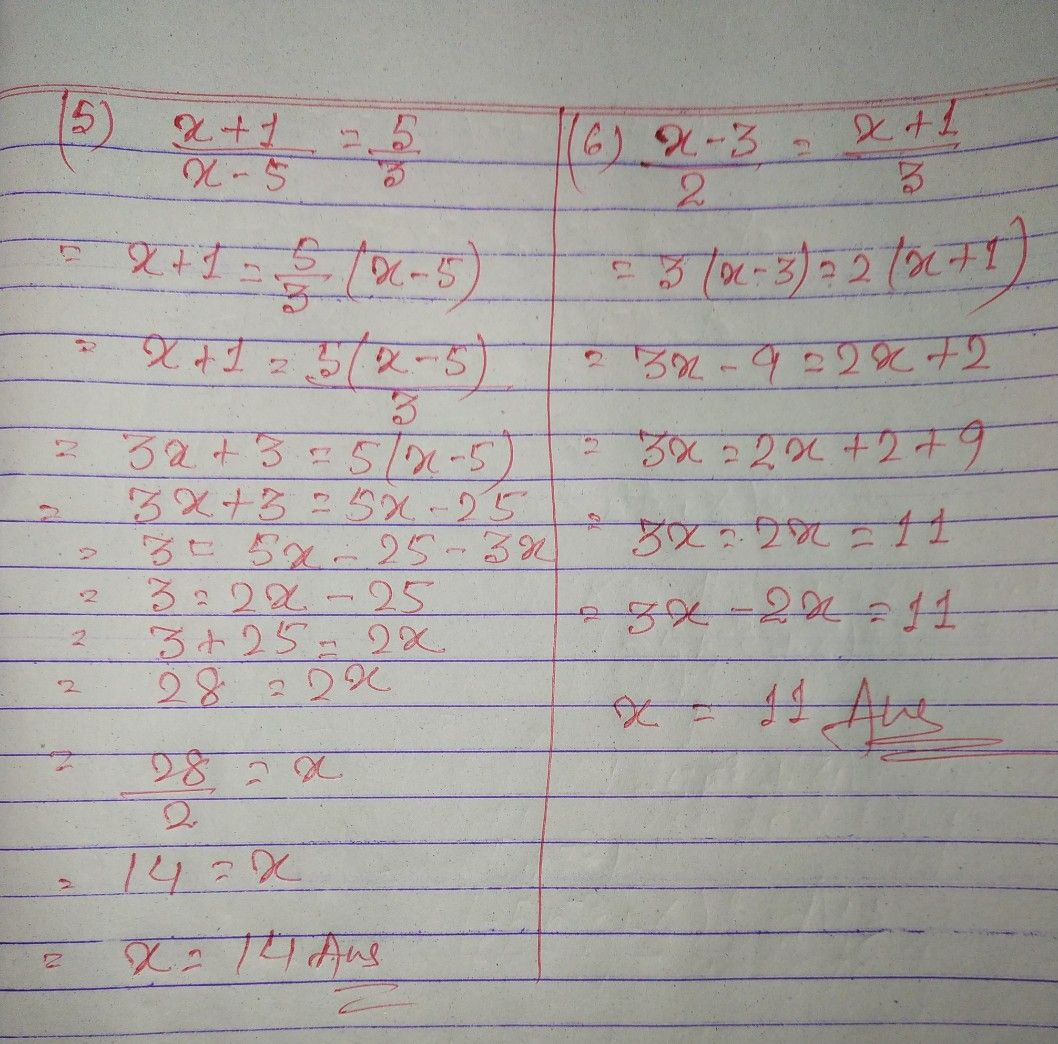Symbol
ProblemActivity $5$ Rational Equations and Inegualities Target $sk111s:$ Solving rational equations and inequalities • Representing $rca1.1if$ • Solving problems rational functions situations using rational functions involving rational equations and inequalities $A$ Solve cach equation and check the solution. $1.$ $\dfrac {y} {9}-\dfrac {2} {5}-\dfrac {1} {3}$ $4$ $\dfrac {x} {6}+\dfrac {3x} {5}=2$ $2$ $\dfrac {x} {5}=15+\dfrac {x} {3}$ $5$ $\dfrac {x+1} {x-5}-\dfrac {5} {3}$ $3.$ $3-\dfrac {2} {x}=\dfrac {4} {x}$ $6$ $\dfrac {x-3} {2}=\dfrac {x+1} {3}$
10th-13th grade
Algebra
Search count: 121
SolutionQanda teacher - Farazkhanwait 2nd page is uploading.....Please evaluate:- 1. Click on top right corner EVALUATE button. 2.Select 5 star. 3.Click on gift coins + three times(C3000)(Not reduce your coins). 4.Click on DONE button on top right corner.Student
thanks ?